• 一直对拉格朗日乘子法不是理解的不是很透彻，今天决定要push一下自己，彻底的理解拉格朗日乘子法，希望对大家有所帮助。 接下来，我将从一下几个方面循序渐进的介绍拉格朗日乘子法： 目录 1 拉格朗日乘子法的...
一直对拉格朗日乘子法不是理解的不是很透彻，今天决定要push一下自己，彻底的理解拉格朗日乘子法，希望对大家有所帮助。

接下来，我将从一下几个方面循序渐进的介绍拉格朗日乘子法：

目录

1 拉格朗日乘子法的基本定义和思想

1.2 拉格朗日乘子法的定义

1.3 KKT（Karush-Kuhn-Tucker）条件

1.3.1 为什么拉格朗日乘子法可以将带约束的优化问题转换成无约束的优化问题?

1.3.2 KKT条件的定义及作用？

2 对偶理论

2.1 原始问题、对偶问题的定义和它们之间的关系

2.2 拉格朗日乘子法中的对偶

2.2.1 对偶函数、原始问题、对偶问题以及一些基本结论

2.2.2 对偶问题与原始问题的关系的推倒

1 拉格朗日乘子法的基本定义和思想

拉格朗日乘子法是一种优化算法，主要用于解决带约束条件下的优化问题。其基本思想就是通过引入拉格朗日乘子，将含有n个变量、k个约束条件的约束优化问题转化为含有（n+k）个变量的无约束优化问题。

1.1 原始问题描述

标准形式的带约束的优化问题可以用如下公式表示: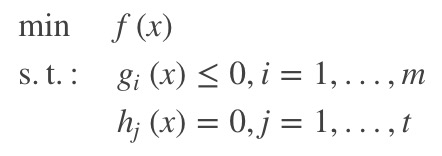公式（1.1）
在公式（1.1）中，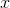是自变量，。该问题的定义域是非空集合为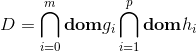, 其中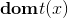表示满足约束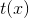的自变量的集合。

为满足约束条件的当前问题的最优解，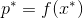为最优解对应的最优值。在当前问题中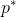为最优极小值。

1.2 拉格朗日乘子法的定义

拉格朗日乘子法的基本思想就是将公式（1.1）中带有约束的最优化求解问题，转换为没有约束的优化问题。它的具体实现如公式（1.2）：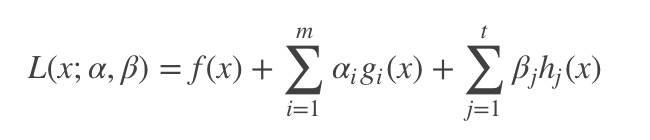公式（1.2）
其中，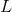是拉格朗日函数，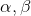就是拉格朗日乗子，且满足。成为primal变量，为dual变量（对偶变量）。

1.3 KKT（Karush-Kuhn-Tucker）条件

本节将详细介绍为什么拉格朗日乘子法可以将带约束的优化问题转换成无约束的优化问题，引出KKT条件的定义并介绍它的含义和作用。

1.3.1 为什么拉格朗日乘子法可以将带约束的优化问题转换成无约束的优化问题?

带约束的优化问题可以分为：等式约束的优化问题和不等式约束的优化问题。接下来，我们将从这两方面进行分析。

1.  等式约束的优化问题。

等式约束的优化问题定义为：假定为维向量，欲寻找的某个取值 ，使目标函数最小，且满足。

可用以下数学公式表示：

公式（1.3）
从几何角度来看，该问题的目标是在由方程确定的维度曲面上寻找能够使目标函数最小化的点。

如图(a)所示，假设自变量包括两个维度。我们不难得出如下结论：

1. 对于约束曲面上的任一点，该点的梯度正交于约束曲面

2. 对于最优点，目标函数在最优点的梯度也正交于约束曲面

由此可知，在最优点，梯度和必然方向相同或者相反。即：存在，使得：

公式（1.4）
其中对应了拉格朗日函数中的拉格朗日乘子。

在该问题中，构造的对应的拉个朗日方程应为：。

不难发现，拉格朗日方程的对于的偏导数 设置为0则等价于公式（1.4）；同时，若将拉格朗日对求得的偏导设置为0，则得到对应的约束条件。

于是，可以证明：拉格朗日乘子法可以将原始的带等式约束的优化问题转化为对拉格朗日函数的无约束优化问题。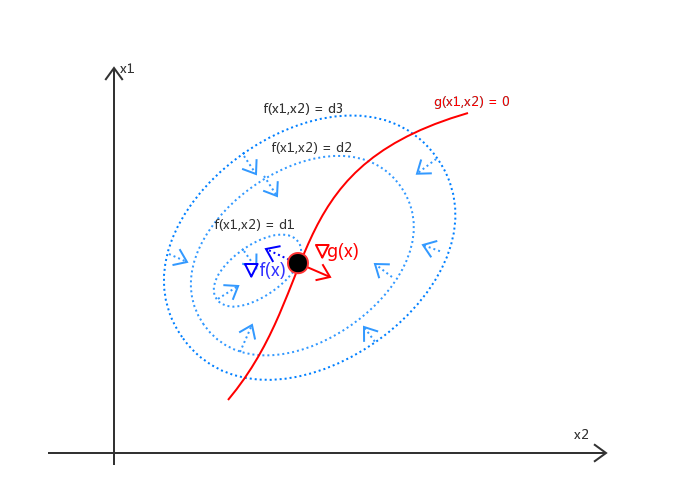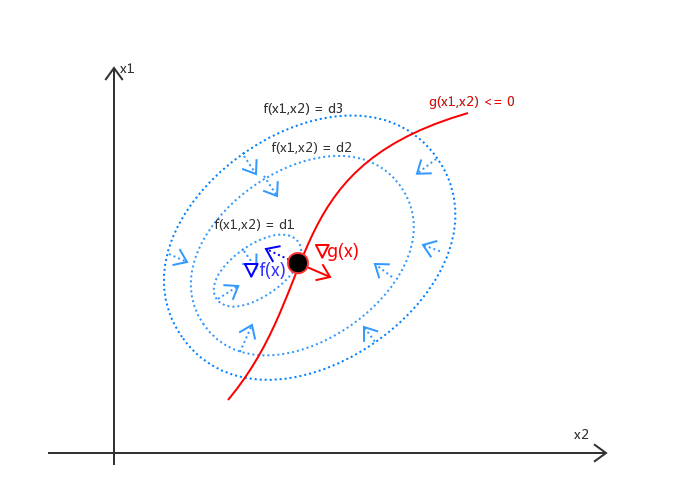(a)  带等式约束的优化问题
(b) 带不等式约束的优化问题

2. 不等式约束的优化问题

现在我们考虑带不等式约束的优化问题。带不等式约束的优化问题可以写成：

公式（1.5）
在该种情况下，最优点的位置只有两种可能：要么存在于约束边界的区域内；要么存在于约束边界上。接下来我们分开分析一下这两种情况。

- 最优点在约束边界区域内

在这种情况下，约束不起作用。我们可以直接通过条件求得最优点。

等价于令后，再对拉格朗日函数求导

- 最优点在约束边界上

这种情况类似于等式约束的优化问题。

但需要注意的是，和必须反向，即存在，使得  。

在整合这两种可能，无论是还是，最优解都必须满足：。

综上可知，拉格朗日乘子法可以将 带不等式约束的优化问题 ，转化成在约束条件（公式（1.6））下对拉格朗日函数求最小化的优化问题。

公式（1.6）
其中公式(1.6)就是KKT条件。

同理，上述做法可以推广到多个约束的情况，即对于1.1节中描述的原始问题，首先我们可以利用拉格朗日乘子法构造拉格朗日方程及其对应的KKT条件：对应KKT条件为：

公式（1.7）
求解1.1节介绍的原始问题等价于求解在满足KKT条件下的拉格朗日函数的最优化的问题。

至此，我们已经证明了拉格朗日乘子法的有效性，它确实可以将原始带约束的优化问题转化为求解满足KKT条件下的拉格朗日函数的最优化问题。

1.3.2 KKT条件的定义及作用？

从上一节的推倒过程我们可知，拉格朗日乘子法可以将带不等式约束的优化问题 ，转化成在KKT条件下对拉格朗日函数求最小化的优化问题。

这句话的含义是，当KKT条件被满足时，对拉格朗日函数的最优化问题才 等价于 原始问题。换句话说，KKT条件是拉格朗日取得可行解的必要条件。只有满足KKT条件，才能求出原始问题的最优解。

2 对偶理论

因为一个优化问题 可以从两个角度进行考察：即：主问题（primal问题）和对偶问题（dual问题）。本节中，我们将首先介绍原始问题、对偶问题及其定关系；然后介绍在带约束的优化问题中对偶函数的定义，以及在拉格朗日乘子法中原始问题的对偶问题是什么、以及对其对偶问题和原始问题的关系的推倒。

2.1 原始问题、对偶问题的定义和它们之间的关系

对偶问题：每一个线性规划问题都伴随有另一个线性规划问题，称为对偶问题。

原始问题（主问题）：原来的线性规划问题则称为原始线性规划问题，简称原始问题。

对偶问题与原始问题之间存在着下列关系：

① 目标函数对原始问题是极大化，对对偶问题则是极小化。

② 原始问题目标函数中的收益系数是对偶问题约束不等式中的右端常数，而原始问题约束不等式中的右端常数则是对偶问题中目标函数的收益系数。

③ 原始问题和对偶问题的约束不等式的符号方向相反。

④ 原始问题约束不等式系数矩阵转置后即为对偶问题的约束不等式的系数矩阵。

⑤ 原始问题的约束方程数对应于对偶问题的变量数，而原始问题的变量数对应于对偶问题的约束方程数。

⑥对偶问题的对偶问题是原始问题，这一性质被称为原始和对偶问题的对称性。

值得注意的是，如果我们能够获得原始问题或者对偶问题的中的任意一个问题的最优解，那么就相当于已经得到另外的问题的最优解。

2.2 拉格朗日乘子法中的对偶

2.2.1 对偶函数、原始问题、对偶问题以及一些基本结论

本节我们先给出对偶函数的定义；然后再介绍拉格朗日乘子法中的原始问题，对偶问题分别是什么。

对偶函数
拉格朗日函数的对偶函数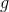的定义：拉格朗日函数关于取得的最小值。可表示为：

公式（2.1）

拉格朗日乘子法中的原始问题、对偶问题分别是什么

原始问题：1.1节给出的带约束的最优化问题

对偶问题：原始问题的对偶问题等于 拉格朗日函数的对偶函数的最优值，即：

公式（2.2）
一些基本结论
结论1：对偶函数构成了原始问题（即公式（1.1））的最优值的下界，即:

公式（2.3）
结论2：若对偶问题和原始问题均有最优解，设对偶问题的最优解对应的最优值为 ，即：

公式（2.4）
其中，的定义为公式（2.2）中给出，它是对偶函数的最优极大值。

2.2.2 对偶问题与原始问题的关系的推倒

接下来我们看一下为什么该结论成立：

结论1证明：

首先，在拉格朗日函数中，对于任意可行点，由于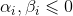，并且 ，，因此有：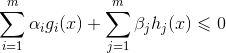公式（2.5）
因而，根据上述不等式，我们可以推出：

公式（2.6）
因此，对任意可行点均有：

公式（2.7）
故而，可以推出公式（2.3）对于的不等式 成立，即：对偶函数是原始问题的最优值的下界。

结论2证明：

从结论1中可知，对偶函数构成了原始问题的最优值的下界，即: 。

故而，对偶函数的全部可能取解，都小于等于原始问题的最优解。

所以，对偶函数的最优值，也一定小于原始问题的最优解。

在目前研究的问题当中，对偶函数具有极大值，那么可以推出结论2：

公式（2.8）
至此，拉格朗日乘子法、对偶问题以及对偶问题和原始问题的关系介绍完毕。

3 拉格朗日乘子法实例

后记：

自参加完一个会议之后，自觉与大牛们差距甚远。往事不可追，后悔之前的荒废时间和不求甚解已经是徒劳。但求以后，以这篇文章为起点，要求自己不论工作亦或是求学都能踏踏实实，全力以赴。十年后再回首不会再有虚度光阴之感。
展开全文对偶问题
这里介绍两个在以后的机器学习算法中经常使用的技巧：拉格朗日乘子（Lagrange multiplier）和梯度下降法（Gradient descent）。

1. 拉格朗日乘子法

拉格朗日乘子被⽤于寻找多元变量在⼀个或者多个限制条件下的驻点。

1.1 等式约束条件

考虑这样一个问题：
求解f(x1,x2)$f(x_1,x_2)$的最大值，其中x1和x2必须满足如下限制条件:g(x1,x2)=0$g(x_1,x_2)=0$。

求解方法1：将g(x1,x2)=0$g(x_1,x_2)=0$转化为x2=h(x1)$x_2=h(x_1)$带入f函数，然后使用微分法求解驻点x∗1$x_1^*$，然后得到驻点x∗2=h(x∗1)$x_2^*=h(x_1^*)$。
这种⽅法的⼀个问题是，把x2显式地表⽰为x1的函数，即找到限制⽅程的解析解很困难。并且，这种⽅法把x1和x2区别对待，这破坏了这些变量之间⾃然存在的对称性。

由此我们引入拉格朗日乘子法。

1.1.1 约束条件g(x)=0$g(x)=0$的特性

设向量x∈RD$x\in R^D$，则g(x)=0$g(x)=0$表示一个D-1维的曲面。
有∇g(x)正交于此曲面\nabla g(x)正交于此曲面

证明： 考虑⼀个位于限制曲⾯上的点x以及这个点附近同样位于曲⾯上的点x + ϵ。如
果我们在点x处进⾏泰勒展开，那么我们有g(x+ϵ)≈g(x)+ϵT∇>g(x)g(x+\epsilon )\approx g(x)+\epsilon ^T\nabla
> g(x) 我们有：g(x+ϵ)=g(x)=0⇒ϵT∇g(x)>≈0g(x+\epsilon )=g(x)=0 \Rightarrow \epsilon ^T\nabla g(x)
> \approx 0ϵ→0⇒ϵT∇g(x)=0\epsilon \to 0 \Rightarrow\epsilon ^T\nabla g(x)=0
ϵ$\epsilon$平行于曲面，所以∇g(x)$\nabla g(x)$正交于曲面。

1.1.2 原问题转化为拉格朗日函数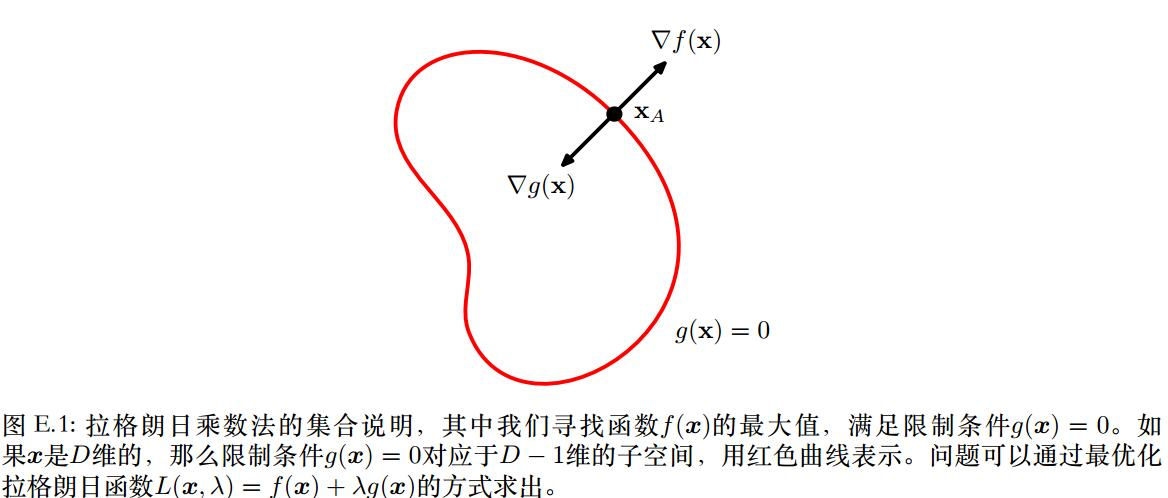在g(x)=0上寻找一个x∗$x^*$,使得f(x)最大。必然有∇f(x∗)$\nabla f(x^*)$正交于限制曲面。

证明： f(x∗+ϵ)≈f(x∗)+ϵT∇f(x∗)f(x^*+\epsilon)\approx f(x^*)+\epsilon ^T\nabla f(x^*)
如果∇f(x∗)$\nabla f(x^*)$不正交于限制曲面，则必然存在ϵ≠0$\epsilon \neq 0$使得ϵT∇f(x∗)>0$\epsilon ^T\nabla f(x^*) \gt 0$，则x∗$x^*$就不再是驻点。

所以对于驻点，∇f//∇g$\nabla f // \nabla g$，所以必然有：∇f+λ∇g=0$\nabla f+\lambda \nabla g=0$。
其中λ≠0$\lambda \neq 0$被称为拉格朗⽇乘数（ Lagrange multiplier）。注意， λ的符号任意。
由此我们定义拉格朗日函数：L(x,λ)=f(x)+λg(x)L(x,\lambda)=f(x)+\lambda g(x)
驻点可由∇xL=0$\nabla_x L=0$求得。

1.2 不等式约束条件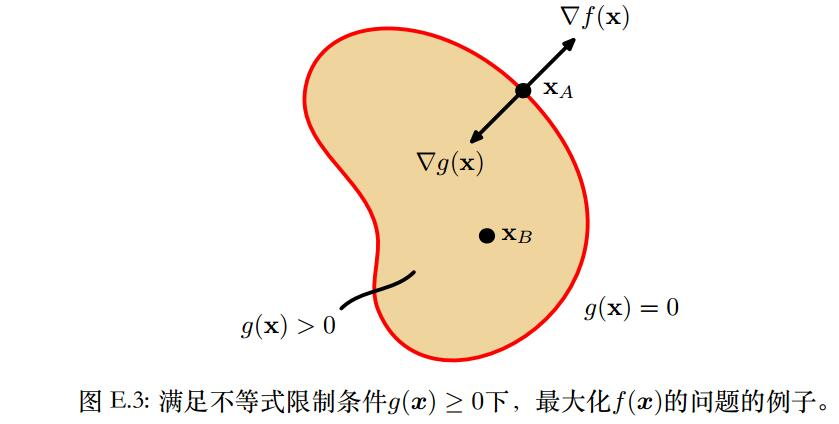考虑在g(x)≥0$g(x) \ge 0$下，f(x)的驻点。

如果驻点位于g(x)>0上，驻点只有一种可能∇f=0$\nabla f=0$，此时g(x)不起作用，也即拉格朗日函数L中λ=0；
如果驻点位于g(x)=0上，那么此问题等同于上述的等式约束条件。有所不同的是，∇f$\nabla f$必然指向g(x)<0$g(x)\lt 0$的方向，也即：对于λ>0，有∇f=−λ∇g$\nabla f=-\lambda \nabla g$。
上述两种情况都会导致λg(x)=0$\lambda g(x)=0$
因此在限制条件g(x) ≥ 0下最⼤化f(x)的问题的解可以通过下⾯的⽅式获得：
L(x,λ)=f(x)+λg(x)L(x,\lambda)=f(x)+\lambda g(x)
限制条件为：
g(x)≥0g(x)\ge 0λ≥0\lambda \ge 0λg(x)=0\lambda g(x)=0被称为KKT条件。

2. 梯度下降法

2.1梯度下降法原理

问题描述：假设f(x),x∈RD$f(x), x\in R^D$上具有一阶连续偏导数，要求解使得f(x)取最小值的点x*。

梯度下降法是一种迭代算法。选取适当的初值x0$x_0$，不断迭代更新x，进行目标函数的最小化，直到收敛。
假设第t次迭代值为xt$x_t$，如何得到xt+1$x_{t+1}$呢？
假设xt+1$x_{t+1}$是xt$x_t$向某一方向v（v是单位向量）移动了η$\eta$长度，则我们希望在给定长度η$\eta$下，选取一个方向，f(xt+1$f(x_{t+1}$下降最多，也即：min||v||=1f(xt+ηv)=f(xt)+ηvT∇f(xt)\min_{||v||=1}f(x_t+\eta v)=f(x_t)+\eta v^T\nabla f(x_t)
我们很容易得到：当方向v与∇f(xt)$\nabla f(x_t)$完全相反时，f(xt+1)$f(x_{t+1})$下降最快。
也即：v=−∇f(xt)||∇f(xt)||v=-\frac {\nabla f(x_t)}{||\nabla f(x_t)||}
这样会产生什么效果呢？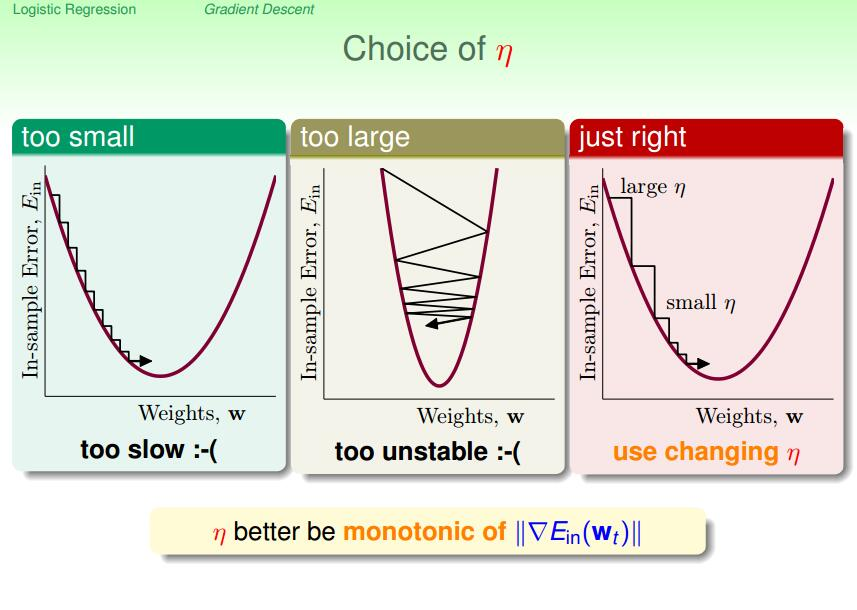当η$\eta$太小时，梯度下降算法会很慢；如果η$\eta$太大，那么算法会不稳定。好的方法是：当梯度比较平缓时，η小一点；当梯度比较陡时，η大一点。也即η与∇f(xt)$\nabla f(x_t)$成正比。
使：η=λ||∇f(xt)||\eta =\lambda ||\nabla f(x_t)||
有：xt+1=xt+ηv=xt+λ||∇f(xt)||⋅−∇f(xt)||∇f(xt)||=xt−λ∇f(xt)x_{t+1}=x_t+\eta v=x_t +\lambda ||\nabla f(x_t)|| \cdot -\frac {\nabla f(x_t)}{||\nabla f(x_t)||}=x_t-\lambda \nabla f(x_t)

2.2 梯度下降法步骤

取初始值x0$x_0$，t=0；
计算f(xt)，∇f(xt)$f(x_t)，\nabla f(x_t)$，如果||∇f(xt)||<ϵ$||\nabla f(x_t)|| \lt \epsilon$，停止迭代，令x∗=xt$x^*=x_t$；否则xt+1=xt−λ∇f(xt)$x_{t+1}=x_t-\lambda \nabla f(x_t)$。
计算f(xt+1)$f(x_{t+1})$，如果||f(xt+1)−f(xt)||<ϵ or ||xt+1−xt||<ϵ$||f(x_{t+1})-f(x_t)||<\epsilon \ or \ ||x_{t+1}-x_t||<\epsilon$时，停止迭代，令x∗=xt+1$x^*=x_{t+1}$。
否则，置t=t+1；转步骤2。

说明：
1. 我们也可以不指定λ，而是每次在步骤2中计算λ，其中λ为：minλ≥0f(xt−λ∇f(xt))$\min_{\lambda \ge 0}f(x_t-\lambda \nabla f(x_t))$。
2. 当目标函数是凸函数时，梯度下降法的解是全局最优解；一般情况下，其解不保证是全局最优解。

2.3 梯度下降的使用策略

2.3.1 Scaling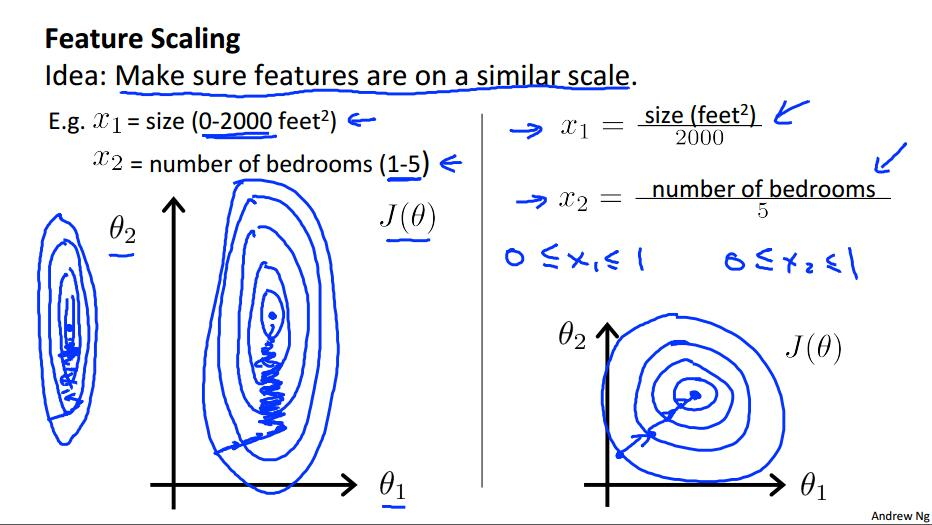如上图，假设有两个feature：x1和x2。如果直接做梯度下降的话，效果如左图：效率会很低。我们通常是将feature变换为−1≤xi≤1$-1 \le x_i \le 1$，当然也没有固定的限制，只要feature大体上变得在同一范围之间即可。大小相差3倍左右是可以接受的。譬如：−3≤xi≤3$-3 \le x_i \le 3$或−13≤xi≤13$-\frac 13 \le x_i \le \frac 13$。
我们经常进行均值归一化：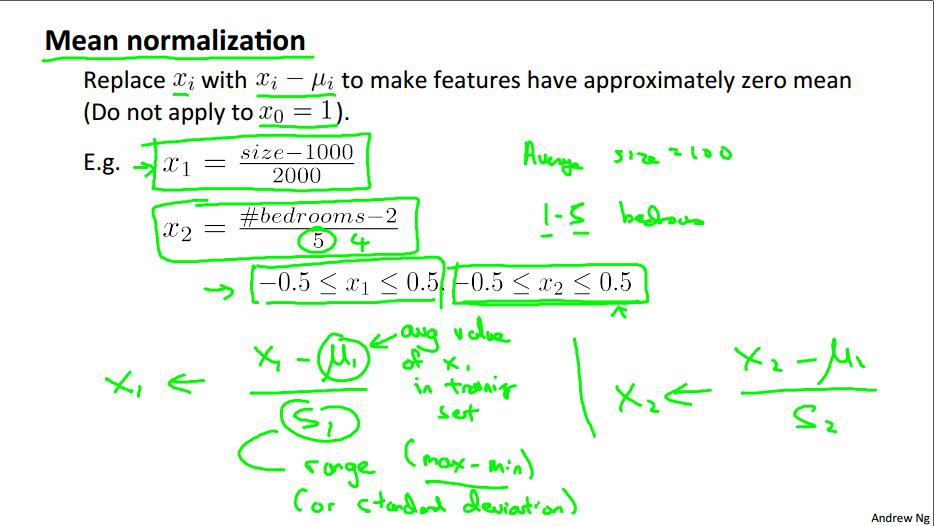2.3.2 确保梯度下降法正确的工作

如何确保其正确的工作，当我们运行梯度下降算法时，我们需要观察Ein$E_{in}$是否随着迭代次数下降：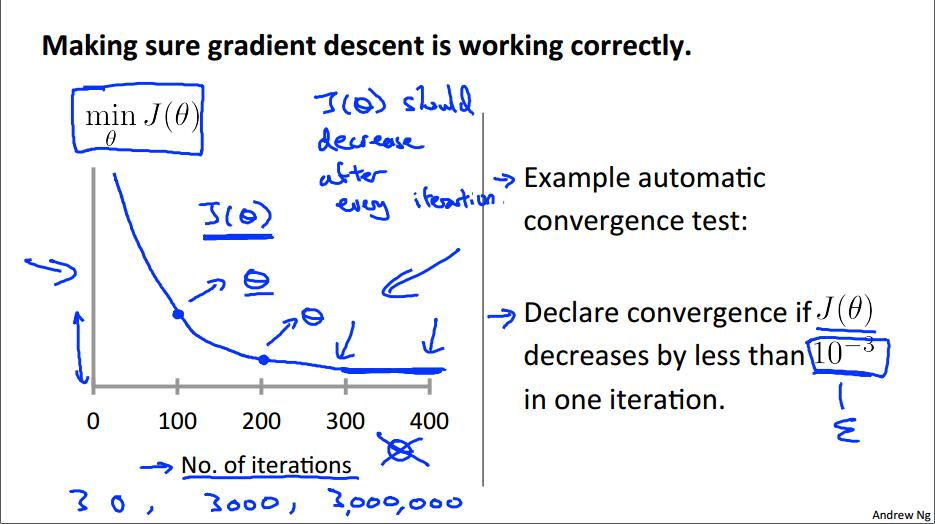J与迭代次数的正确关系如上图所示，我们可以通过上图确定合理的迭代次数。如果需要迭代次数太多，也即下降太慢，则说明学习速率太低，需要用更大的学习速率。

那么异常情况是什么呢？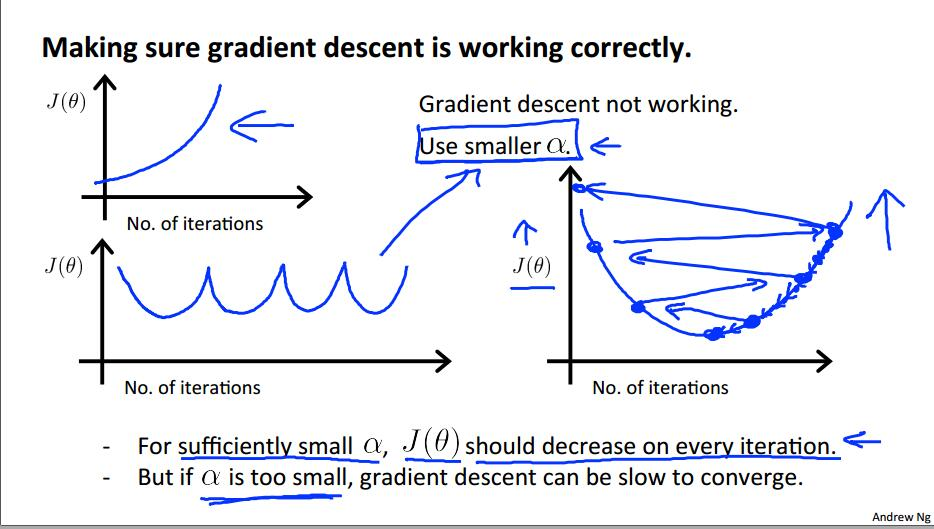如上图，损失函数J并不随着迭代次数递减，这说明学习速率过大，需要用更小的学习速率。

通常情况下，我们会试验多个不同的学习速率λ，选择合适的学习速率：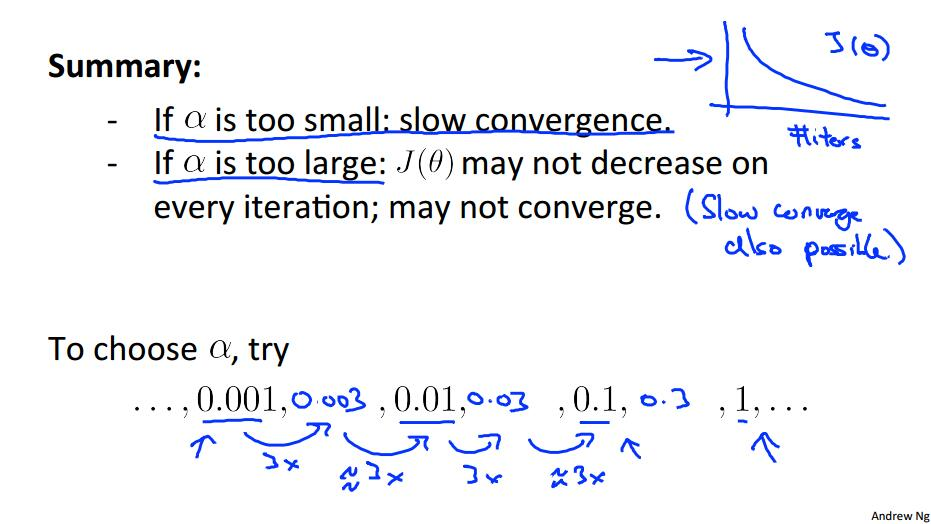2.4 梯度下降的其他变式

2.4.1 随机梯度下降法

考虑如下算法：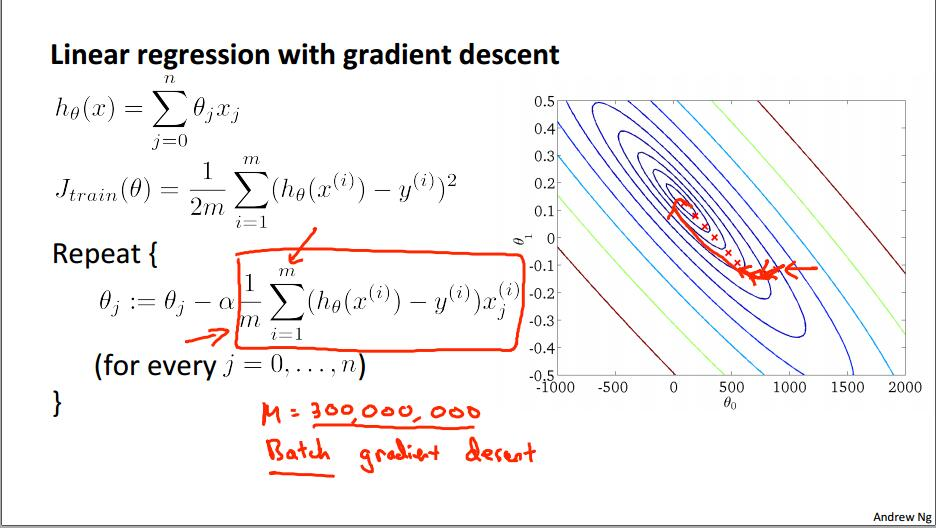我们使用梯度下降算法进行线性回归。假设training set有3亿数据，那么每次迭代，对于每一个特征参数，都需要对3亿个数据同时进行运算，这样做的效率太低。

我们仔细观察上式中梯度1m∑mi=1(h(x(i)−y(i))x(i)$\frac 1m \sum_{i=1}^m(h(x^{(i)}-y^{(i)})x^{(i)}$，它是由m个数据平均而来的，我们设∇j(θ,x(i),y(i))=(h(x(i)−y(i))x(i)\nabla j(\theta, x^{(i)},y^{(i)})=(h(x^{(i)}-y^{(i)})x^{(i)}将所有的m个点视为整体，则有：∇J(θ)=E(∇j(θ,x(i),y(i)))\nabla J(\theta)=E(\nabla j(\theta, x^{(i)},y^{(i)}))这一关系就是随机变量中样本点与期望之间的关系，有：∇j(θ,x(i),y(i))=∇J(θ)+variance\nabla j(\theta, x^{(i)},y^{(i)})=\nabla J(\theta)+variance我们完全可以随机选择一个点来近似梯度，而不是每次计算都使用所有点：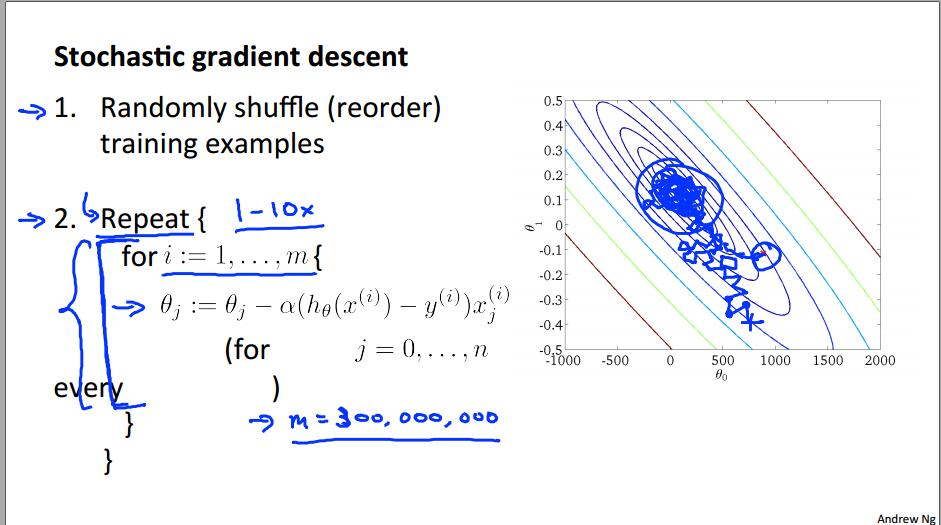2.4.2 Mini-batch 梯度下降法

根据随机梯度的思路，我们很容易的就能有mini-batch梯度的想法：
在梯度计算中，将m视为总体，是随机选取某个样本来估计∇J(θ)$\nabla J(\theta)$好，还是选取b个样本来估计∇J(θ)$\nabla J(\theta)$好？根据Hoeffding定理很容易得出结论。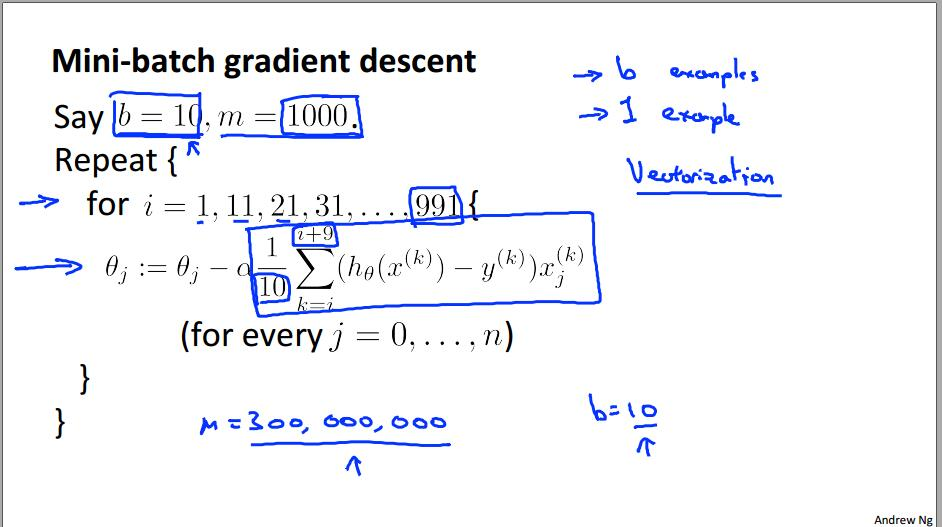展开全文机器学习
• 机器学习拉格朗日对偶性 @(Machine Learning) 学习SVM的时候，解决最优化问题需要应用到拉格朗日对偶性，现在总结下相关概念。 Reference： 《统计学习方法》 附录C_拉格朗日对偶性 李航 本文完全根据该...
【机器学习】拉格朗日对偶性

@(Machine Learning)

学习SVM的时候，解决最优化问题需要应用到拉格朗日对偶性，现在总结下相关概念。

Reference：
《统计学习方法》  附录C_拉格朗日对偶性 李航
本文完全根据该附录总结，内容基本一致，有条件的请阅读该附录原版。

原始问题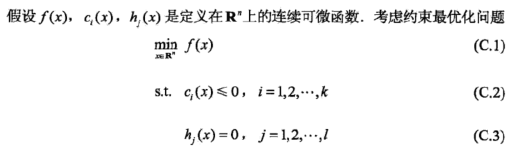引入广义拉格朗日函数：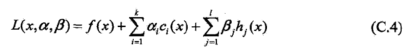考虑将之最大化：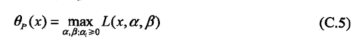如果给定x，x违反原始问题的约束条件：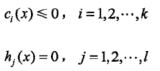有：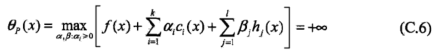原因不难，如下：
如果x违反条件，
则存在某个ci(x)> 0，令ai->+∞，最大可得+∞；
或存在某个hj(x)!= 0，令βjhj(x)->+∞，最大可得+∞。

如果x满足条件，则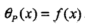综上有：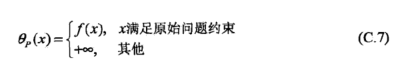为了使x满足条件，我们最小化该函数。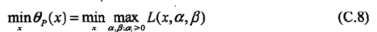容易得到该函数和原始最优化问题是等价的。
我们称这个问题为广义拉格朗日函数的极小极大问题。

对偶问题

定义：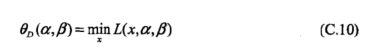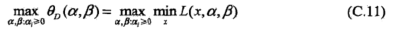上述问题称为广义拉格朗日函数的极大极小问题。
表示为约束最优化问题：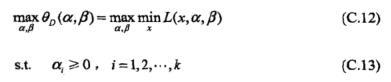该问题称为原始问题的对偶问题。

原始问题与对偶问题的关系

定义原始问题的最优值：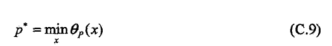定义对偶问题的最优值：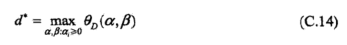有如下定理：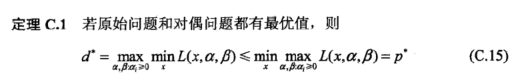意思是：对偶问题的最优值小于等于原始问题的最优值。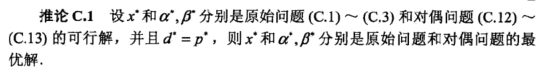意思是：某些条件下，原始问题和对偶问题最优值相等，这时候可以用解对偶问题替代原始问题。
可得下面定理：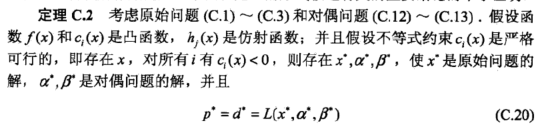最后得出最重要的定理，证明不展开：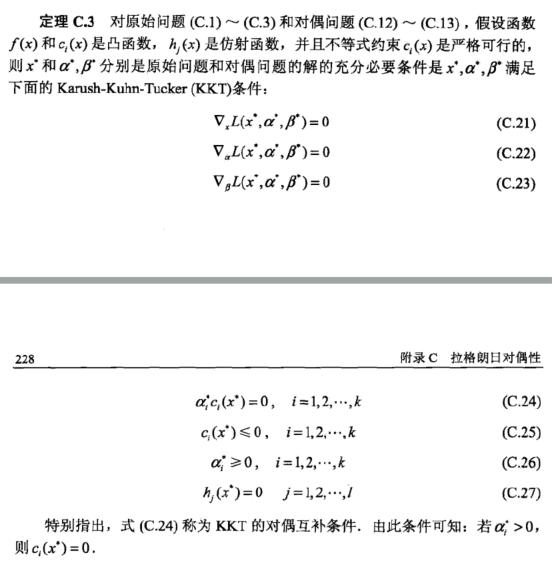简单来说就是如果我们想要通过对偶问题得到原始问题的解，需要满足KKT条件。
展开全文• 以下内容摘自周志华老师的《机器学习拉格朗日乘子法是一种寻找多元函数在一组约束条件下的极值的方法，通过引入拉格朗日乘子，可将有d个变量与k个约束条件的最优化问题转换为具有d+k个变量的无约束优化问题...

以下内容仅供参考，如有错误，欢迎指出

文章目录什么是拉格朗日乘子法预备知识——水平集只具有一个等式约束的凸函数最优化问题问题描述问题的解决方案拉格朗日乘子法为什么有效的简单推导只具有一个不等式约束的凸函数最优化问题问题描述问题的解决方案拉格朗日乘子法为什么有效的简单推导多等式约束与多不等式约束的凸函数最优化问题问题描述问题的解决方案
什么是拉格朗日乘子法
以下内容摘自周志华老师的《机器学习》

拉格朗日乘子法是一种寻找多元函数在一组约束条件下的极值的方法，通过引入拉格朗日乘子，可将有d个变量与k个约束条件的最优化问题转换为具有d+k个变量的无约束优化问题求解

预备知识——水平集
以下内容摘自安全学求过

在数学领域中, 一个具有n变量的实值函数f的水平集是具有以下形式的集合
$\{(x_1,...,x_n) | f(x_1,...,x_n) = c \}$
其中 c 是常数. 水平集即使得函数值具有给定常数的变量集合.
当具有两个自变量时, 称为水平曲线(等高线), 如果有三个自变量, 称为水平曲面, 更多自变量时, 水平集被叫做水平超曲面.

只具有一个等式约束的凸函数最优化问题
本节暂时只讨论求解满足等式约束条件下，凸函数最小的情况，但其求解方法同样适用于使目标函数最大的情况。
问题描述
假定x为d维向量，欲寻找x的某个取值$x^*$，使凸函数$f(x)$最小并满足$g(x^*)=c$，c为常数，即：
$\min \limits_{x} f(x)\\ s.t \ g(x)=c$

问题的解决方案
使用拉格朗日乘子法,具体步骤如下

定义拉格朗日函数$L(x,\lambda)=f(x)+\lambda(g(x)-c) \ (\lambda!=0) \ (式1.0)$
计算\left\{ \begin{aligned} \frac{\partial L(x,\lambda)}{\partial x}=0\\ \frac{\partial L(x,\lambda)}{\partial \lambda}=0 \end{aligned} \right.
d+1个变量(x是d维向量，有d个变量，加上一个$\lambda$)，d+1个方程，一定可以进行求解，求解出的$x$代入$f(x)$

拉格朗日乘子法为什么有效的简单推导
为便于理解，我们在三维函数上进行讨论，即利用拉格朗日乘子法解决以下凸优化问题：
$\min \limits_{x,y} f(x,y)\\ s.t \ g(x,y)=c$
我们在xOy平面上画出函数$f(x,y)$的等高线和函数$g(x,y)$的等高线（概念见预备知识一节中的水平集），接着，我们只保留函数$g(x,y)=c$时的等高线，结果如下图：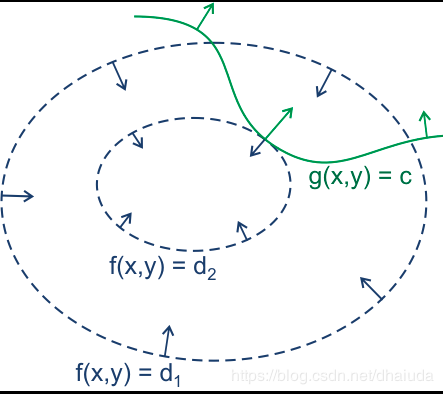上图的蓝色箭头表示函数$f(x,y)$梯度的反方向，绿线表示函数$g(x,y)$的取值为c时的梯度方向，(x,y)只能位于绿线上，现在我们要求位于绿线上的点对应的$f(x,y)$的最小值，我们来思考一下

这里先理解一下登高线形成的圈有什么含义，对于凸函数来说，等高线形成的圈越小，则离全局最小越接近，现在我们有一个想法，$g(x,y)=c$的等高线与函数$f(x,y)$的若干等高线有若干交点，每个交点所处的等高线围成的圈大小不同，圈越小，对应的$f(x,y)$取值越小，那么只需要找到圈最小处的交点，不就是函数$f(x,y)$在$g(x,y)=c$约束下的最小值了吗？

那么圈最小处的交点有什么特性呢？注意到圈最小，意味着该圈内部的圈不可能在与函数$g(x,y)=c$的登高线具有交点，否则这个圈就不是最小了，因为外层的圈肯定比内层的圈要大，这意味圈最小处的交点位于的等高线与函数$g(x,y)=c$相切

相切意味着什么？这里有一个定理——梯度与登高线的切线垂直，证明请查看为什么梯度的方向与等高线切线方向垂直
由此可知，相切意味着两个函数的梯度共线，即存在常数$\lambda$满足$\nabla f(x,y)=\lambda \nabla g(x,y)$，即$\nabla f(x,y)-\lambda \nabla (g(x,y)-c)=0$(为什么要减c下面解释)，将负号统一到$\lambda$中即得到$\nabla f(x,y)+\lambda \nabla (g(x,y)-c)=0$，即函数$L(x,y,\lambda)=f(x,y)+\lambda (g(x,y)-c)$（式1.1）对x，y求偏导，故有
$\frac{\partial f(x,y)}{\partial x}+\lambda \frac{\partial (g(x,y)-c)}{\partial x}=0\\ \frac{\partial f(x,y)}{\partial y}+\lambda \frac{\partial (g(x,y)-c)}{\partial y}=0$
式1.1具有三个变量，但是只有两个方程，我们还差一个方程，不怕不怕，式1.1对$\lambda$求导，即得到约束条件，与上述两个方程联立，此时即可求解出x、y、$\lambda$。

式1.1之所以多减去一个c，是为了让式1.1对$\lambda$求导置0得到约束条件，将相切的点限制在$g(x,y)=c$中（$g(x,y)$与$f(x,y)$等高线相切的点有很多），此时将约束问题统一为对式1.0的无约束问题（只需将式1.0对$x、y、\lambda$求导即可）

值得注意的是，相切处并不一定就是最小值，也有可能是最大值，即函数等高线形成的圈内部相切于约束等高线，因此我们需要将求得的多个相切值代入原式，从而取最小

只具有一个不等式约束的凸函数最优化问题
问题描述
假定x为d维向量，欲寻找x的某个取值$x^*$，使凸函数$f(x)$最小并满足$g(x^*)\leq 0$，即：
$\min \limits_{x} f(x)\\ s.t \ h(x) \leq 0$

问题的解决方案
使用拉格朗日乘子法,具体步骤如下

定义拉格朗日函数$L(x,\lambda)=f(x)+\lambda h(x) \ (\lambda!=0) \ (式1.2)$
计算\left\{ \begin{aligned} \frac{\partial L(x,\lambda)}{\partial x}=0\\ \frac{\partial L(x,\lambda)}{\partial \lambda}=0 \end{aligned} \right.
求出的$x、y、\lambda$需要满足KKT条件，否则不是原问题的解，KKT条件即为\left\{ \begin{aligned} & h(x) \leq 0 \\ & \lambda \geq 0 \\ &\lambda h(x)=0 \end{aligned} \right.
上述过程在多维凸函数的多不等式约束求极值问题中依然能够成立

拉格朗日乘子法为什么有效的简单推导
考虑不等式约束$h(x)$，我们分两种情况讨论

凸函数的极值位于$h(x)< 0$处，此时不等式约束根本不起作用，即式1.2的$\lambda=0$，直接求解$\nabla f(x)=0$即可
凸函数的极值不位于$h(x)<0$处，如下图(阴影部分表示$h(x)<0$，箭头表示梯度的逆方向)：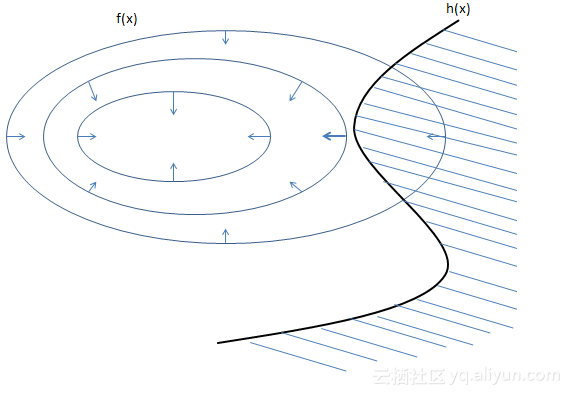此时变为凸函数在$h(x)=0$下的极值问题，但此时有一个隐含条件 —— $f(x)$与$h(x)$的梯度方向相反，意味着$\lambda>0$，理由如下：
由于凸函数$f(x)$的极值不在$h(x)<0$处，$f(x)$在切点的梯度方向将指向上图阴影部分（因为最小值不在阴影部分内，对切点来说，意味着凸函数$f(x)$在阴影部分的点取值比不在阴影部分的点取值要大），而函数$h(x)$在切点处的梯度方向指向阴影外部，因为阴影内部的点满足$h(x)<0$，切点满足$h(x)=0$，而梯度指向增长最快的方向，则$h(x)$在切点的梯度方向指向外部

当$\lambda=0$时，为第一种情况，当$\lambda>0,g(x)=0$时，为第二种情况，以上两种情况综合起来，就是KKT条件，此时仍然有许多约束条件，我们可以使用拉格朗日对偶对其进行求解，请查看机器学习——SVM预备知识 拉格朗日对偶推导与证明

多等式约束与多不等式约束的凸函数最优化问题
问题描述
假定x为d维向量，欲寻找x的某个取值$x^*$，使凸函数$f(x)$最小并满足$g(x^*)\leq 0$，即：
$\min \limits_{x} f(x)\\ s.t \ h_i(x) \leq 0 （i=1,2,3....n）\\ \ \ \ \ g_j(x)=0 （j=1,2.....m）$

问题的解决方案
将上两节的内容进行推广即可得到拉格朗日乘子法解决多等式约束与多不等式约束的凸函数最优化问题的形式
$L(x,\lambda,\mu)=f(x)+\sum_{i=1}^m\mu_ig_i(x)+\sum_{i=1}^n\beta_ih_i(x)$
需满足
\left\{ \begin{aligned} &g_i(x) \leq0 & i=1,2.....n\\ &\mu_i \geq0 & i=1,2.....n\\ &\mu_ig_i(x)=0 & i=1,2.....n\\ &h_j(x)=0 &j=1,2.....m\\ &\frac{\partial L(x_k,\mu,\beta)}{\partial x_k}=0 &k=1,2....d \end{aligned} \right.
约束多的可怕，不怕不怕，通过拉格朗日对偶可以转换为非常简介的形式，请查看机器学习——SVM预备知识 拉格朗日对偶推导与证明


展开全文• 文章目录什么是插值预备知识拉格朗日插值法牛顿插值法 什么是插值 摘自百度百科 在离散数据的基础上补插连续函数，使得这条连续曲线通过全部给定的离散数据点 本节博客介绍拉格朗日插值法与牛顿插值法 预备知识 ...

以下内容均为个人理解，如有错误，欢迎指出

文章目录什么是插值预备知识拉格朗日插值法牛顿插值法牛顿插值法与拉格朗日插值法的应用参考文献
什么是插值
摘自百度百科

在离散数据的基础上补插连续函数，使得这条连续曲线通过全部给定的离散数据点

本节博客介绍拉格朗日插值法与牛顿插值法

预备知识

对于一个二维平面上的离散点，若其满足函数的定义，即一个x，只对应一个y，则可以唯一确定一个多项式函数

为了证明上述结论，我们需要证明这个多项式函数的存在性与唯一性，设n次多项式函数为$y=a_nx^n+a_{n-1}x^{n-1}+....+a_0$，平面上存在n个不同的离散的点，记为$(x_1,y_1),(x_2,y_2),...,(x_n,y_n)$，这n个点满足函数的定义，则有：$y_1=a_nx_1^n+a_{n-1}x_1^{n-1}+....+a_0\\ y_2=a_nx_2^n+a_{n-1}x_2^{n-1}+....+a_0\\ ......\\ y_n=a_nx_n^n+a_{n-1}x_n^{n-1}+....+a_0$
用矩阵表示上述所有式子得
$\begin{bmatrix} y_1\\ y_2\\ .\\ .\\ y_n \end{bmatrix} =\begin{bmatrix} 1 \ x_1 \ x_1^{2}...\ x_1^{n}\\\\ 1 \ x_2 \ x_1^{2}...\ x_2^{n}\\ ....\\ 1 \ x_1 \ x_1^{2}\ ...\ x_n^{n}\\ \end{bmatrix}* \begin{bmatrix} a_0\\ a_1\\ ....\\ a_n \end{bmatrix}（式1.1）$
令$A=\begin{bmatrix} 1 \ x_1 \ x_1^{2}...\ x_1^{n}\\\\ 1 \ x_2 \ x_1^{2}...\ x_2^{n}\\ ....\\ 1 \ x_1 \ x_1^{2}...\ x_n^{n}\\ \end{bmatrix},X=\begin{bmatrix} a_0\\ a_1\\ ....\\ a_n \end{bmatrix},Y=\begin{bmatrix} y_1\\ y_2\\ .\\ .\\ y_n \end{bmatrix}$
则有$AX=Y$,只需求出$X$，过这n个点的多项式就求出来了，$A$是著名的范德蒙矩阵，当$x_i,1 \leq i \leq n$两两不相等时，其行列式不等于0，意味着给定$Y$，$X$具有唯一解，由此可知，二维平面上过所有已知点的多项式函数有且仅有一个
有了上述结论，我们来想一想应该如何求解式1.1，我们只需要构造一个n次多项式，使得这个多项式在$x=x_n$时，$y=y_n$即可，拉格朗日和牛顿从两个不同的角度解决了这个问题，这两个方法构造的多项式函数都是相同的

拉格朗日插值法
拉格朗日构造的n次多项式如下：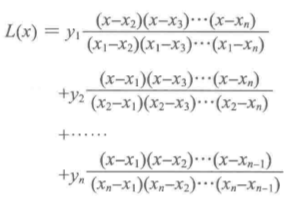注意上式满足$L(x_1)=y_1,L(x_2)=y_2,.....,L(x_n)=y_n$
拉格朗日插值法固然简单，但是每当出现新的离散点时，整个多项式都需要重新构造，为了解决这个问题，我们有了牛顿插值法

牛顿插值法
拉格朗日插值法是从已知的全部点出发，求得多项式函数的，因此当出现多的点时，需要重新计算一遍，那我们可不可以用递推的方式来构造多项式呢？即已知过n-1个点的多项式函数，在此基础上对其进行更改，使其过第n个点，由此一来，当出现多的点时，就不需要重新构造一遍多项式函数。这就是牛顿插值法的出发点。
那么问题就是如何在已知过n-1个点的多项式函数$f_{n-1}(x)$的基础上，构造出过n个点的多项式函数表达式，牛顿给出的答案是：$f_n(x)=f_{n-1}(x)+b_n(x-x_1)(x-x_2)....(x-x_{n-1})（式1.2）$
注意上式，当$x=x_i$ ，$1 \leq i \leq n-1$，$f_n(x_i)=f_{n-1}(x_i)=y_i$ (由于多项式函数$f_{n-1}(x)$过前n-1个点)
那么现在的问题是如何求解$b_n$，这个简单，只需要将第n个点代入其中即可求解，那么$b_n$解的形式有没有什么规律呢？幸运的是，在式1.2的形式下，$b_n$的解可以表示n阶均差的形式，即$f[x_n,x_{n-1},.....,x_1]=\frac{f[x_n,x_{n-1},.....,x_2]-f[x_{n-1},.....,x_1]}{x_n-x_1}$
而n阶均差可以通过递推的方式求得，例如当n=5时，有：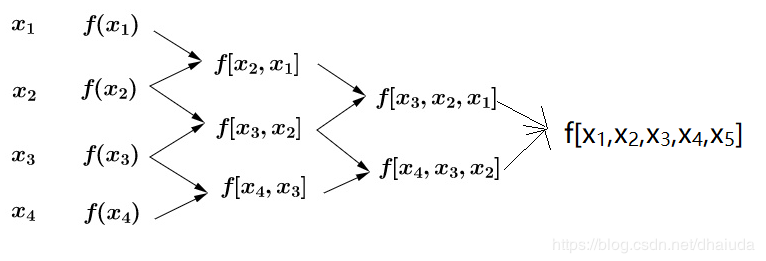一阶均差可以直接求得，例如$f[x_2,x_1]=\frac{f(x_2)-f(x_1)}{x_2-x_1}$
对式1.2进行展开，我们得到牛顿插值法的表现形式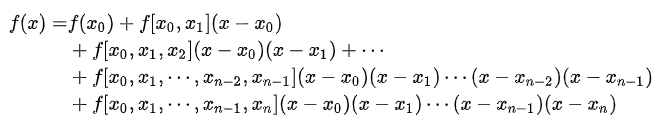当出现新的点$(x_{n+1},f(x_{n+1}))$时，只需要通过上面描述的阶差的递推方式求出$b_{n+1}$即可获得过这n+1个点的多项式函数，例如我们以有过四个点的多项式函数，现在多出一个点$(x_5,f(x_5))$，我们通过下列方式求$b_5$: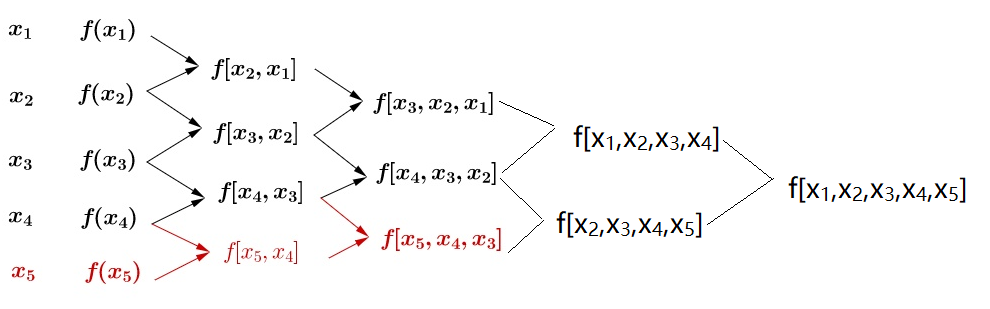根据式1.2便可求得过这5个点的多项式函数

牛顿插值法与拉格朗日插值法的应用
牛顿插值法与拉格朗日插值法可以用于缺失值的填充

参考文献
1、维基百科插值
2、牛顿插值的几何解释是怎么样
3、如何直观地理解拉格朗日插值法？


展开全文• 在数学最优问题中，拉格朗日乘数法（以数学家约瑟夫·路易斯·拉格朗日命名）是一种寻找变量受一个或多个条件所限制的多元函数的极值的方法。这种方法将一个有n 个变量与k 个约束条件的最优化问题转换为一个有n + k...拉格朗日定理 机器学习
• 在约束最优化问题中，常利用拉格朗日乘子法将原始问题转换为对偶问题求解。即通过引入拉格朗日乘子，将有ddd个变量和kkk个约束条件的最优化问题转化为具有d+kd+kd+k个变量的无约束优化问题求解。这种方法的最典型...拉格朗日乘子法
• 1.引言本篇博客主要总结了拉格朗日乘子和KTT条件在机器学习中求解最优值的原理，博主尽量举点小例子帮助大家一起共同学习。2.拉格朗日和KTT作用我们在求解问题时，经常会遇到一些在约束条件下求解函数的。 在有等式...拉格朗日乘子 机器学习
• 以下内容在周志华老师《机器学习》的基础上加以理解而成，首先先明白，SVM之所以引入拉格朗日对偶，是为了降低算法复杂度，并且引入核函数时，在数学上更加自然，因此在本文的推导过程中，会发现不等式约束越来越多...
•机器学习 拉格朗日乘数法
• 这个问题我们之前涉及到过,在机器学习实战(基于scikit-learn和TensorFlow)学习心得(23)--逻辑回归 Logistic Regression的时候我们把带约束条件的方程通过拉格朗日乘子法合并为了一个方程. 拉格朗日乘子法具体是什么...线性代数 机器学习
•  机器学习是一门多领域交叉的学科，是人工智能的核心，其应用遍及人工智能的各个领域，专门研究计算机是如何模拟或实现人类的学习行为，以获取新的知识或技能，重新组织已有知识结构，使之不断改善自身的性能。...机器学习 机器学习算法 机器学习实战 python机器学习
• 上一节讲到SVM的优化公式，并提到SVM在强大的数学理论背景之下有着十分高效的训练方法，本节就先来讲讲在这之中的一个关键知识点——拉格朗日乘子法，为之后深入讲解SVM做准备。机器学习 拉格朗日乘子法 SVM 支持向量机
• 首先需要明确的是拉格朗日乘子法是一种凸优化算法，所以目标函数是一个凸函数，约束条件也是一个凸函数。 1.带有等式约束的最小值问题  （这是约束条件） 求 的极小值. 目标函数在三维空间中是一个倒立的...机器学习 拉格朗日乘子法 对偶问题
• 凸优化和拉格朗日函数 对偶问题和拉格朗日函数： 拉格朗日函数对于乘子是线性的或者放射的。 对x属于域中的所有函数值取下界，就剩下了关于 λ 和 υ 的函数。 拉格朗日函数值是小于我们假设函数最有解的。...机器学习 凸优化 拉格朗日函数
• 机器学习中常见的优化方法： 梯度下降法、牛顿法拟牛顿法、共轭梯度法、拉格朗日乘数法 主要内容 梯度下降法 牛顿法拟牛顿法 共轭梯度法 拉格朗日乘数法   许多机器学习算法，往往建立目标函数（损失...
• 1.1蛇形环形矩阵 import pprint import numpy as np def main(): N = int(input('请输入数字：')) myarray = np.zeros((N, N), dtype=np.int16) x, y = 0, N - 1 res = myarray[x][y] = 1 ...蛇形矩阵 机器学习
• 本达人课针对机器学习初学者，从机器学习、深度学习最基本的原理及学习意义入手，以模型为驱动，带领大家吃透几个最经典的机器学习模型——学习这些模型的原理、数学推导、训练过程和优化方法。 本课为每个模型提供...人工智能 机器学习
• 学习了一个学期机器学习算法，从什么都不懂到对十个机器学习算法有一定的了解，下面总结一下十大机器学习算法，从算法的概念、原理、优点、缺点、应用等方面来总结，如果有错误的地方，欢迎指出。 目录 1.决策树...
• 拉格朗日乘子法无疑是最优化理论中最重要的一个方法。但是现在网上并没有很好的完整介绍整个方法的文章。所以小编整理了如下文章，希望能博得大家一赞。 在求取有约束条件的优化问题时，拉格朗日乘子法（La...
...